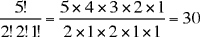Quandaries and Queries My name is Amanda and I am actually a college Freshman, my prob. and stat. teacher gave us this problem and then figured out he didn't know how to work it. How many 6 digit numbers can you make from the numbers 0,0,2,2,4,4, giving that 0 cannot come first. The number has to contain 2 twos, 2 fours, and 2 zeros. He says the answer in the teachers manual is 60 but he was not sure how they got that. Is that the answer and if so I did you get it? Hi Amanda, Since the first digit can't be 0 you have two choices, 2 or 4. Now you are left with 5 digits 0,2,2,4 and 4 which can go in any of the 5 remaining positions. The number of ways of arranging 5 objects, 2 of one kind, 2 of another kind and 1 of a third kind isThus the number of 6 digit numbers you can form from ,0,2,2,4,4 if 0 cannot come first is 230=60. Cheers, Penny A second solution, Line out the 6 spots open for the digits: _ _ _ _ _ _ Next, select the 2 spots where you will put the zeroes: These have to be among the 5 rightmost spots, so you have 2 spots to choose among 5. There are 5*4/2 = 10 ways to do this. Next, select 2 spots in the remaining 4 where you will put the twos. There are 4*3/2 = 6 ways to do this. Your number is now entirely determined: the fours go in the remaining 2 spots. In all, there were 10*6 = 60 ways to select spots for the zeroes and then select spots for the twos. Claude Go to Math Central